Advanced Multimedia Processing Lab -- Projects -- Rate Shaping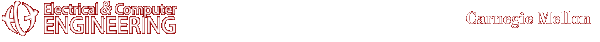#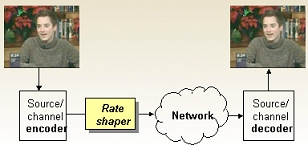Trista Pei-chun Chen peichun@andrew.cmu.edu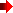Top of this page

 Motivation and Goal

Is it a dream to watch the news clip/newest movie trailer while you are out of the office? To overcome the high error rate of the wireless channel as well as utilizing the bandwidth intelligently, we use rate shaping on coded video bitstream to deliver the video content effectively.Top of this page

Joint Source/Channel Coding with Rate Shaping

The proposed system of joint source-channel coding with rate shaping is shown as follows in Figure 1.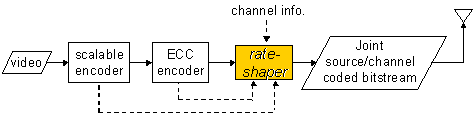(a) Encoder/Sender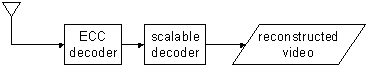(b) Decoder/Receiver

Figure 1. System overview of the joint source/channel coder. (a) sender; (b) receiver

The video bitstream to be shaped is coded in multiple layers, using scalable coding, followed by unequal error correction coding (ECC) in each layer.  If each layer of the scalable coded video bitstream is protected by a segment of error correction codes, there are D (D: number of scalable coding layers) scalable coding segments and D error correction coding segments for the rate shaper to choose from to send to the wireless network. For example, if there are 2 layers of scalable coding, there are a total of 6 combinations of segments for the rate shaper to choose from. That is, the combinations (x, y) = {(0, 0), (1, 0), (1, 1), (2, 0), (2, 1), (2, 2)} are valid. The first entry being the number of scalable coding segments chosen and second entry being the number of error correction coding segments chosen. We can optimize the selection of the rate shaping by examining the discrete rate distortion map of these combinations as follows: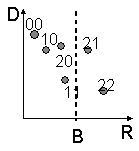Figure 2. Rate distortion map example. D: distortion, R: rate, B, bandwidth requirement

Discrete Rate Distortion Combination

We further improve the system by smartly allocating the bandwidth budgets across coding units. The bandwidth allocation is accomplished by the discrete rate distortion combination algorithm illustrated below: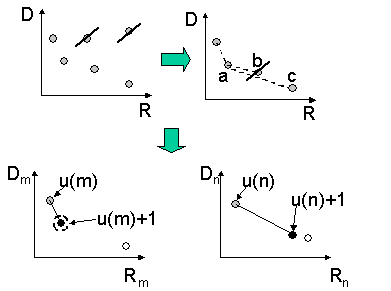Figure 3. Discrete rate distortion combination algorithm

Experiment

Figure 4 compares the rate shaping results of the conventional method by Vass and Zhuang with the proposed rate shaping method using discrete rate distortion combination algorithm.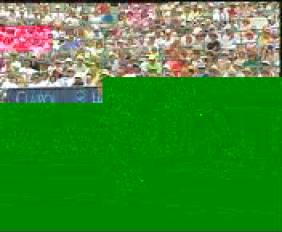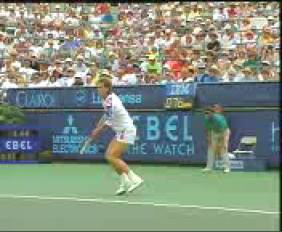(a) (b)

Figure 4. Sample frames of rate shaping results. (a) Vass and Zhuang's; (b) the proposed

Path-Based Rate Shaping

The path-constrained rate shaping can be applied for efficient multicast.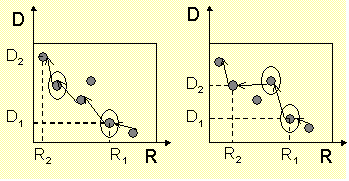Figure 5. Path-constrained rate shapingTop of this pageTop of this pageTop of this page

Any suggestions or comments are welcome. Please send them to Trista Pei-chun ChenTop of this page